Total marks: --
Total time: --
INSTRUCTIONS
(1) Assume appropriate data and state your reasons
(2) Marks are given to the right of every question
(3) Draw neat diagrams wherever necessary

1 (a) Compare open loop and closed loop control system and give one practical example of each.
6 M
1 (b) For the system shown in below, write mechanical network and obtain its mathematical model.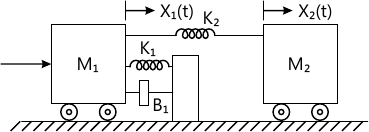6 M
1 (c) For the system shown in below, Write its mechanical network and obtain mathematical model and electrical analogue based on force-current analogy.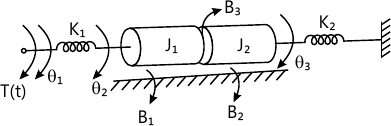8 M

2 (a) Define transfer function and what are its properties.
5 M
2 (b) Obtain the transfer function for the block diagram shown in below, using block diagram reduction method.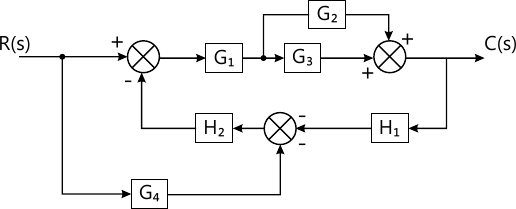7 M
2 (c) For the block diagram, given in below, obtain overall transfer function using Mason's gain formula.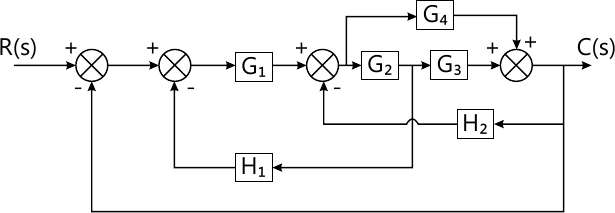8 M

3 (a) Draw the time response curve and define time domain specifications, for second order C.S. for unit step i/p.
6 M
3 (b) A unity feedback control system is given by an open-loop transfer function, $G(s)= \dfrac {K}{s(s+10)}$ i) The value of K for ?=0.5
For this value of K tr=? and Mp=? for unit step input.
6 M
3 (c) The open loop transfer function of servo system with unity feedback is given by $G(s)=\dfrac {10}{s(0.ls+1)}$ Find out static error constant and obtain steady state error when subjected to an i/p of $r(t)=A_0+A_1t+\dfrac {A_2}{2}t^2$
8 M

4 (a) Explain RH stability criterion used for finding of stability of control systems.
6 M
4 (b) Find the range of K for the system to be stable using RH criterion $G(s)H(s)= \dfrac {k(1-s)}{s(s^2+5s+9)}$
6 M
4 (c) Investigation stability of the system given by characteristics equation
S2+2S5+8S4+12S3+20S2+16S+16=0.
8 M

5 A feedback control system has an open loop transfer function $G(s)H(s)= \dfrac {K}{s(s+3)(S^2+2s+2)}$ Draw the root locus as K varies from 0-to-?
20 M

6 (a) Define the following terms:
i) Resonant peak
ii) Resonant frequency
iii) Band width
iv) Cut off frequency
4 M
6 (b) Sketch the bode plot for the transfer function $\dfrac {300 (s^2+2s+4)}{s(s+10)(s+20)}$
13 M
6 (c) Write a note about gain margin in brief.
3 M

7 (a) Plot the polar plot for the transfer function given $G(s)=\dfrac {1}{s(Ts+1)}$
8 M
7 (b) State Nyquist stability criterion verify stability of the system described below:
$G(s)H(s) = \dfrac {5}{s(1-s)}$
12 M

8 (a) Obtain the state model for the electrical system given below. Take e1(t), e2(t) as i/p variables and voltage across R as o/p variables.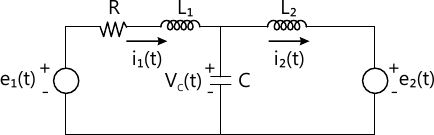8 M
8 (b) List out the properties of STM.
5 M
8 (c) Obtain the state transition matrix for a system matrix given by $A=\begin{bmatrix}0 &1 \\-2 &-3 \end{bmatrix}$
7 M

More question papers from Control Systems